Maxwell’s Equations: Poynting Theorem

# Maxwell’s Equations: Poynting Theorem - Notes | Study Electromagnetic Fields Theory (EMFT) - Electrical Engineering (EE)

 1 Crore+ students have signed up on EduRev. Have you?

Potential Formulation of Maxwel’s Equations

We have arrived at the set of Maxwell’s equations, which are,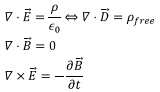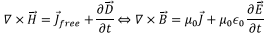along with the constitutive relations,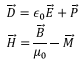In this lecture will will attempt a formulation of the problem in terms of potentials. It may be observed that Maxwell’s equations are four equations for six quantities, viz., the components of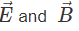Let us recall that in electrostatics, we had defined a scalar potential by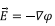and in magnetostatics we introduced a vector potential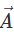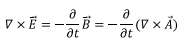which gives us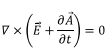Notice that since with the introduction of Faraday’s law, the electric field did not remain conservative, we have not replaced the electric field with the gradient of a scalar function. We have still retained a vector potential because the magnetic field was a general vector field and it can always be expressed as a curl of yet another vector. However, the vector potential would not be the same as it was in the magnetostatic case.

Since the curl of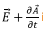is zero, this combination is conservative and being an irrotational field can be expressed as the gradient of a scalar function V. We define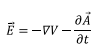Let us see what this does to the Maxwell’s equations.

In vacuum, we have,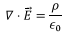In terms of V and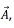we have, on taking the divergence of both sides of the preceding equation,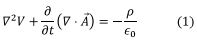which contains both the electrostatics Gauss’s law and the Faraday’s law.

Let us consider the remaining pair of Maxwell’s equations. The divergence of magnetic field continues to be zero even after introduction of time varying electric field. However, Ampere’s law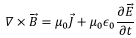can be restated as follows: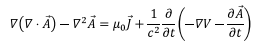where we have used,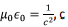c being the speed of light in vacuum, a universal constant.

We can rewrite this equation, by rearranging terms, as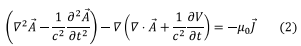Equations (1) and (2) are two equations in four quantities (three components of A and one component of V) which are equivalent to the four Maxwell’s equations that we had obtained. These equations are not decoupled.

We will now use a choice of gauge that we have for the potential. We know that the scalar potential V is undetermined up to a constant while we can add gradient of a scalar function to the vector potential.

We will use,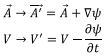subject to Lorentz Gauge condition,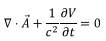the equations (1) and (2) get decoupled and give a pair of inhomogeneous wave equatons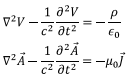solutions of which can always be found. However, can we ensure that Lorentz gauge can always be satisfied? The answer is yes. Suppose we have a pair of V and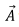for which Lorentz gauge is not satisfied and we have,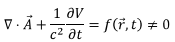where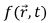is a scara function of space and time. Let us define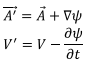In terms of these, we have,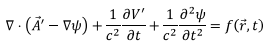We can get Lorentz gauge to be satisfied A' for and V' if we have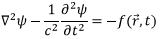which is equation for which a solution can always be found. Thus Lorentz gauge condition can always be satisfied.

Energy Density of electromagnetic field

Let us calculate the energy density of electromagnetic field and see how the energy contained in such field can change in a closed volume.

we have seen that the energy of a collection of charges can be written as follows: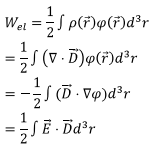where in the penultimate step, we have used the relation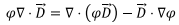and converted the integral of the first term on the right to a surface integral by the divergence theorem and discarded the surface integral by taking the surface to infinity so that the remaining integral is all over space. The electric energy density can be thus written as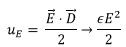the last relation being true for a linear electric medium.

Likewise, the magnetic energy can be written as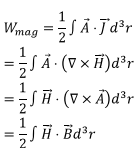The magnetic energy density is given by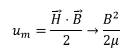the last relation is valid for a linear magnetic medium.

The total energy density in electromagnetic field is thus given by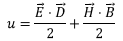Poynting Theorem

Consider the case of an electromagnetic field confined to a given volume. How does the energy contained in the field change? There are two processes by which it can happen. The first is by the mechanical work done by the electromagnetic field on the currents, which would appear as Joule heat and the second process is by radiative flow of energy across the surface of the volume.

The mechanical power is given by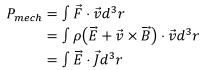where the work done by the magnetic field is zero. This amount of energy appears as Joule heat.

The rate of change of energy in the volume can be calculated as follows. For a linear electric and linear magnetic medium,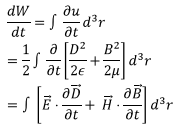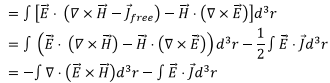Define “Poynting Vector”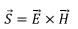We have,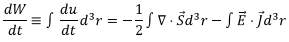As the relation is valid for arbitrary volume, we have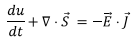This equation represents the energy conservation equation of electrodynamics. Converting the term representing volume integral of the Poynting vector to a surface integral, we get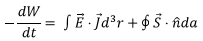where we have denoted the surface element by da instead of the usual dS so that it does not cause confusion with our notation for the Poynting vector.

The second term on the right is the rate of flow of energy across the boundary of the region. We will see later that similar conservation law is valid for momentum contained in the field as well.

Tutorial Assignment

1. A long straight wire of resistivity ρ and cross sectional area Acarries a steady current I. Calculate the amount of energy that enters a unit length of the wire.
2. A long solenoid of radius R and having n turns per unit length has a core of permeability μ. The current in the turns of the solenoid is increasing at a uniform rate. Find the energy contained per unit length of the solenoid and express it in terms of the self inductance of the wire. What is the amount of energy flowing through the unit area of the surface of the solenoid?

Solutions to Tutorial Assignments

1. The energy enters through the surface of the wire and hence we are interested in the fields on the surface of the wire. By Ampere’s law, the magnetic field is given by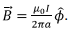The electric field is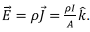Thus the Poynting vector is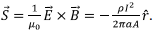The amount of energy entering the surface per unit length of the wire is (ds is area element on the surface)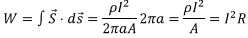where R is the resistance per unit length of the wire. The electromagnetic field provides the energy to compensate for the Joule loss in the wire.

2. The magnetic field is axial and is given by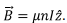The corresponding induced electric field is obtained from Faraday’s law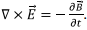The induced current and the electric field must be circumferential,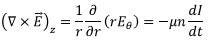which gives,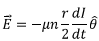The Poynting vector, points radially and is to be calculated on the surface of the solenoid (as it has no component on the end faces of the solenoid)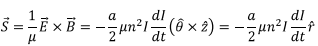The energy flowing through the surface for a length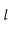of the solenoid is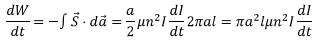The total energy density is given by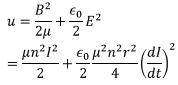so that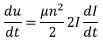the electric field term is dropped because the current is changing at a constant rate. Integrating over the volume, the rate of energy flow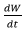can be seen to be given by the same expression as obtained above. Comparing this with the expression for the self inductance of a solenoid,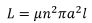we get,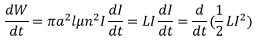Self Assessment Questions

1. A charge q is moving with a uniform velocity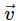. Obtain its Poynting vector and show that the energy propagates along with the moving charge.

2. A parallel plate capacitor with circular plates of radius R is being charged at a uniform rate by current I in an external circuit. Obtain an expression for the Poynting vector when it is being charged. Calculate the flow of energy through imaginary cylindrical surface defined by the two plates of the capacitor.

Solutions to Self Assessment Questions

1. The electric field due to the charge is given by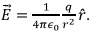A moving charge can be considered as a current,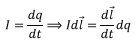which gives,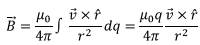as the integrand does not depend on q. Thus, the Poynting vector is given by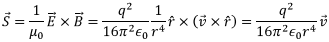because the radial direction is perpendicular to the direction of velocity.

2. The magnetic field was calculated in the last lecture in terms of the displacement current, it is given by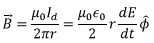The electric field is directed from the positive plate to the negative plate (defined to be z direction) and is written as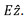The Poynting vector is given by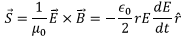The rate of energy flow through the imaginary cylindrical surface is obtained by integrating the Poynting vector and is given by (since the surface points inward)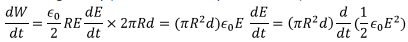the last relation shows that the energy flow is at the cost of the energy stored in the electric field.

The document Maxwell’s Equations: Poynting Theorem - Notes | Study Electromagnetic Fields Theory (EMFT) - Electrical Engineering (EE) is a part of the Electrical Engineering (EE) Course Electromagnetic Fields Theory (EMFT).
All you need of Electrical Engineering (EE) at this link: Electrical Engineering (EE)

## Electromagnetic Fields Theory (EMFT)

11 videos|46 docs|62 tests
 Use Code STAYHOME200 and get INR 200 additional OFF

## Electromagnetic Fields Theory (EMFT)

11 videos|46 docs|62 tests

Track your progress, build streaks, highlight & save important lessons and more!

,

,

,

,

,

,

,

,

,

,

,

,

,

,

,

,

,

,

,

,

,

;